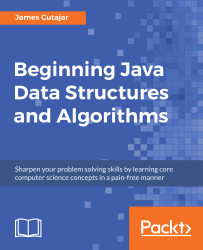•#### Beginning Java Data Structures and Algorithms#### Overview of this book

Learning about data structures and algorithms gives you a better insight on how to solve common programming problems. Most of the problems faced everyday by programmers have been solved, tried, and tested. By knowing how these solutions work, you can ensure that you choose the right tool when you face these problems. This book teaches you tools that you can use to build efficient applications. It starts with an introduction to algorithms and big O notation, later explains bubble, merge, quicksort, and other popular programming patterns. You’ll also learn about data structures such as binary trees, hash tables, and graphs. The book progresses to advanced concepts, such as algorithm design paradigms and graph theory. By the end of the book, you will know how to correctly implement common algorithms and data structures within your applications.
Title PagePackt UpsellContributorsPrefaceFree Chapter
Algorithms and ComplexitiesSorting Algorithms and Fundamental Data StructuresHash Tables and Binary Search TreesString Matching AlgorithmsGraphs, Prime Numbers, and Complexity ClassesOther Books You May EnjoyIndex## Chapter 1. Algorithms and Complexities

An algorithm is a set of logical instructions to perform a particular task. Algorithms are everywhere nowadays. As a software developer, understanding the core principles of algorithms and data structures will enable you to make informed decisions on how to approach a particular problem. This is valid whether you're working in a bank writing accounting software or doing medical research data, mining genetic code. How do we determine which is the right algorithm to use when more than one solution to a problem exists? In this chapter, we will examine different types of algorithms and discuss how the performance varies in each. We will discuss what makes an algorithm more efficient than another and how to express the complexity of each.

### Note

The common examples of algorithms include traffic lights regulating congestion on the streets, face recognition software on smartphones, recommendation technologies, and so on. It's important for you to understand that an algorithm is just a small part of an application used to solve a well-defined problem. Examples such as sorting a list of numbers, finding the shortest route, or word prediction are all correct. Big software applications, such as email clients or an operating system are improper examples.

By the end of this chapter, you will be able to:

• Define an algorithm with an example
• Measure algorithmic complexity
• Identify algorithms with different complexities
• Assess various examples with different runtime complexities